# Big-O Notation

## Big-O Notation in Data Structure

In this article, I am going to discuss Big-O Notation in Data Structure. Please read our previous article where we discussed the Analysis of the Algorithm and why it is important to analyze the algorithm. At the end of this article, you will understand what is Big-O Notation and how to calculate the time complexity of an algorithm using the Big-O Notation in Data Structure.

##### What is Big-O Notation?

Big-O Notation is a symbol or we can say it is a symbolic notation which says that how your algorithm is performed if the input data changes. mostly when the input data increases. What it means. When we talk about the algorithm, algorithms have three pillars. The first one is the Input, the second one is the processing which takes place by the algorithm. And the final one is the output. For better understanding, please have a look at the following diagram. Big-O Notation tells that if your input data increases, then how and in what rate, your algorithm processing time increases. So, it actually tells you the relationship between your processing time and the increase of data.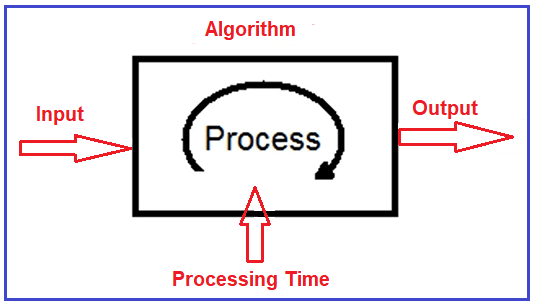##### Example:

Let us understand the above Big-O Notation definition with an example. Let say you have an algorithm. In that algorithm, let’s assume for 5 records it takes approximately (not exactly) 27 seconds and if we increase the records to 10, it takes 105 seconds and if we further increase the records to 50, it takes approximately 2550 seconds. For better understanding, please have a look at the following image.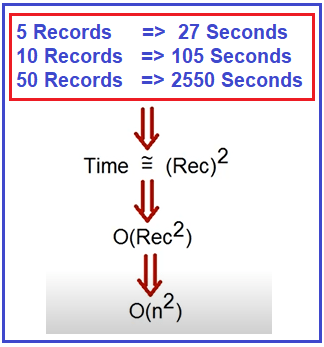If you see here the relationship, the time required to process by this algorithm is the number of records squares. So, we can represent the time with the letter big O and simply replace records with n. Here, n is nothing but the input data. So, we can represent this with O(n2). So, when we say O(n2), it means that the processing time required by that algorithm will be the square of the data input.

#### Programming Examples to understand Big-O Notation:

##### Example1: O(1)

The following method does not do anything great. It simply accepts a list if string as input and returns the first element from the collection. In the following example, if we increase the input data, then also the processing time will not change. The processing time is fixed. If you pass 100 lists of strings or event thousands list of strings, the time required to fetch the first element is almost the same. The symbolic way of representing this function or algorithm is O(1). O(1) means the algorithm performance will not change when the input data changes.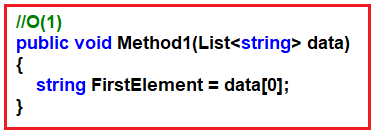Note: The performance will constant irrespective of the input size.

##### Example: O(n)

In the following example, the method takes a list of strings as input and then tries to find a particular element from the collection using a for each loop. In this case, when the number of strings increases the processing time is also going to increase. For example, for 10 input strings if it takes x time, when the number of records increases, the number of for loop increases, and accordingly the processing time is also increased. Here, the processing time is directly proportional to the number of input elements. The symbolic way of representing this function or algorithm is 0(n). O(n) means as the number of input increases the processing time also increases.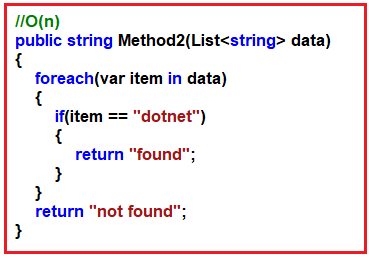##### Example3: O(2n) or O(n2)

In the following example, we are using a double for each loop. For each record in the first loop, we will loop each record in the second loop. This algorithm will take twice the time of the records. The symbolic way of representing this function or algorithm is 0(2n). It also possible this can be O(n2).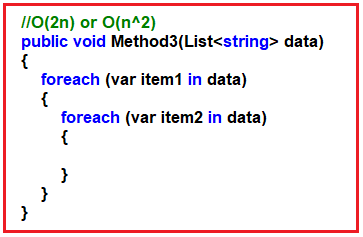##### Big-O Notation Graphical Representation:

So, when we see a Big-O notation symbol, we can classify how that algorithm is going to work from the performance point of view. For better understanding, please have a look at the following diagram which shows the relationship between the time and the number of records.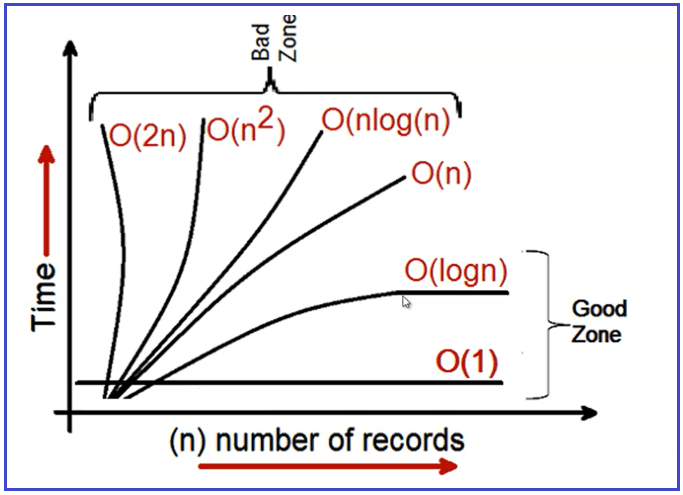O(1): As we already discussed it is constant. Even if the number of records or input data increases, the processing time will be constant. When you see O(1), then you should not be a worry as it is a good algorithm.

O(logn): From a practical point of view, definitely when the number of records increases, there will be a performance drop. If there is a performance drop, we will be happy to see O(logn). In O(logn), initially, the performance decrease, but at some point in time, the performance is going to the same. Here, for the first 100 records, it may take 100ms, for 200 records it may take 200ms, but from 500 records the time is going to be minimal.

Note: O(1) and O(logn) are considered as good gone.

O(n): It is linear. When we see O(n), then we need to worry about the algorithm. When the number of data is increasing or the input is increasing, the processing time is also increasing linearly. It is considered an OK zone.

O(2n)/O(n2)/O(nlogn): When you see expositional or quadratic, then it is considered to be a bad zone. Generally, we don’t want to see the performance of the algorithm in these zones.

Note: So based on the Big-O Notation, you can identify your algorithm is in which zone. Whether it is in a good zone, or Ok zone, or a bad zone and you can think accordingly.